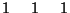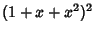## Trinomial Triangle

The Number Triangle obtained by starting with a row containing a single 1'' and the next row containing three 1s and then letting subsequent row elements be computed by summing the elements above to the left, directly above, and above to the right:(Sloane's A027907). Theth row can also be obtained by expandingand taking coefficients:and so on.

Sloane, N. J. A. Sequence A027907 in The On-Line Version of the Encyclopedia of Integer Sequences.'' http://www.research.att.com/~njas/sequences/eisonline.html.